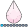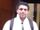## Create new variable with a combination of categories

I'm creating a new variable test_result_all based on test_result_1, test_result_2, and test_result_3. Each variable contains 4 categories: positive, negative, indeterminate, and Not Done.

For any positive result in the 3 variables, test_result_all='positive'. For any test result with negative but not positive, the result is negative. For all results with Not Done=Not Done. For all results with indeterminate=indeterminate. Otherwise (mixed between indeterminate and Not Done, or missing), it's missing.

How should I code that? I was thinking of doing an if-then but it seems complicated to do it.

I started the following but couldn't figure out what's next:

if test_result_1='P' or test_result_2='P' or test_result_3='P' then test_result_all='P';

else if...

1 ACCEPTED SOLUTION

Accepted SolutionsHB
Barite | Level 11

## Re: Create new variable with a combination of categories

Starting point:

``````data have;
input TR1:\$1. TR2:\$1. TR3:\$1.;
cards;
P P P
P N N
N N N
N P N
M M M
I I I
D D D
D I M
;
run;

data want;
set have;
trall = 'M';
if (tr1='P' or tr2='P' or tr3='P') then trall = 'P';
if (tr1='N' or tr2='N' or tr3='N') and (tr1 ne 'P' and tr2 ne 'P' and tr3 ne 'P')  then trall = 'N';
if (tr1='I' and tr2='I' and tr3='I') then trall = 'I';
if (tr1='D' and tr2='D' and tr3='D') then trall = 'D';
run;

``````

Yields

 The SAS System TR1 TR2 TR3 trall P P P P P N N P N N N N N P N P M M M M I I I I D D D D D I M M
6 REPLIES 6novinosrin
Tourmaline | Level 20

## Re: Create new variable with a combination of categories

Hi, May i request you to please post your question with a sample HAVE dataset and a sample WANT dataset. I am apologetically too lazy to write it down. I prefer copy/paste. Thank you!HB
Barite | Level 11

## Re: Create new variable with a combination of categories

Starting point:

``````data have;
input TR1:\$1. TR2:\$1. TR3:\$1.;
cards;
P P P
P N N
N N N
N P N
M M M
I I I
D D D
D I M
;
run;

data want;
set have;
trall = 'M';
if (tr1='P' or tr2='P' or tr3='P') then trall = 'P';
if (tr1='N' or tr2='N' or tr3='N') and (tr1 ne 'P' and tr2 ne 'P' and tr3 ne 'P')  then trall = 'N';
if (tr1='I' and tr2='I' and tr3='I') then trall = 'I';
if (tr1='D' and tr2='D' and tr3='D') then trall = 'D';
run;

``````

Yields

 The SAS System TR1 TR2 TR3 trall P P P P P N N P N N N N N P N P M M M M I I I I D D D D D I M M

## Re: Create new variable with a combination of categories

It worked! Thank you very much!

## Re: Create new variable with a combination of categories

@jcapua2 wrote:

I'm creating a new variable test_result_all based on test_result_1, test_result_2, and test_result_3. Each variable contains 4 categories: positive, negative, indeterminate, and Not Done.

For any positive result in the 3 variables, test_result_all='positive'. For any test result with negative but not positive, the result is negative. For all results with Not Done=Not Done. For all results with indeterminate=indeterminate. Otherwise (mixed between indeterminate and Not Done, or missing), it's missing.

How should I code that? I was thinking of doing an if-then but it seems complicated to do it.

I started the following but couldn't figure out what's next:

if test_result_1='P' or test_result_2='P' or test_result_3='P' then test_result_all='P';

else if...

You say 4 categories:  positive, negative, indeterminate, and Not Done but then reference missing. That makes 5 categories.

You also say those values but then post code testing for a value of 'P'. So which are the actual values of the categories?

Else if should be similar to the test for 'P' but use the value for negative.

You would then have further "else if".

If I understand "For all results with Not Done=Not Done. For all results with indeterminate=indeterminate" then instead of 'or' the requirement would be to use 'and' between all of the tests similar to your example.

If none of the above is true then the result will be missing. No need to create an explicit assignment but it would look like:

else test_result_all='';

or

else call missing(test_result_all);PGStats
Opal | Level 21

## Re: Create new variable with a combination of categories

Why do you test test_result_1='P' if each variable contains 4 categories: positive, negative, indeterminate, and Not Done? It can never be true.

PG

## Re: Create new variable with a combination of categories

Let's assume your codes are single letters code, with letters 'P' (for positive), 'N' (negative), 'D' (not done), or 'I' (indeterminate).

And you want:

1. If there is at least 1 'P' the overall result is 'P'
2. otherwise if there is at least 1 'N' --> 'N'
3. otherwise if you have three 'D's or three 'I's then the overall result is correspondingly a 'D' or 'I'
4. otherwise missing

If that's correct, then collapse your three codes into a single 3-letter code  as in

three_letters=cats(test_result1,test_result2,test_result3);

Then you can use the index function to good effect.  For example

if index(three_letters,'P')>0 then test_result_all='P';

``````data have (drop=i j k);
array tr {3} \$1 test_result1-test_result3;
do i='P','N','D','I'; tr{1}=i;
do j='P','N','D','I'; tr{2}=j;
do k='P','N','D','I'; tr{3}=k;
output;
end;
end;
end;
run;

data want;
set have;
three_letters=cats(test_result1,test_result2,test_result3);

if index(three_letters,'P')>0 then test_result_all='P'; else
if (some condition) then test_result_all='N'; else
if (some condition) then test_result_all='D'; else
if (some condition) then test_result_all='I';
run;``````

Print out the resulting list of three_letters and test_result_all to see if it does what you want.

`` ``

--------------------------
The hash OUTPUT method will overwrite a SAS data set, but not append. That can be costly. Consider voting for Add a HASH object method which would append a hash object to an existing SAS data set

Would enabling PROC SORT to simultaneously output multiple datasets be useful? Then vote for
Allow PROC SORT to output multiple datasets

--------------------------
Discussion stats
• 6 replies
• 1090 views
• 4 likes
• 6 in conversation